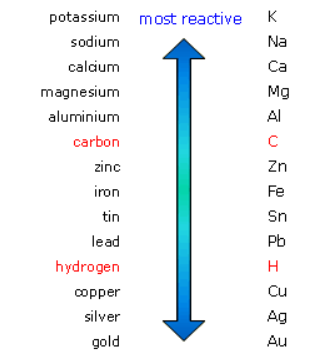×
Get Full Access to Introductory Chemistry - 5 Edition - Chapter 16 - Problem 18q
Get Full Access to Introductory Chemistry - 5 Edition - Chapter 16 - Problem 18q

×

# Are metals at the top of the activity series the easiest or hardest to oxidize?ISBN: 9780321910295 34

## Solution for problem 18Q Chapter 16

Introductory Chemistry | 5th Edition

• Textbook Solutions
• 2901 Step-by-step solutions solved by professors and subject experts
• Get 24/7 help from StudySoup virtual teaching assistantsIntroductory Chemistry | 5th Edition

4 5 1 337 Reviews
24
0
Problem 18Q

Are metals at the top of the activity series the easiest or hardest to oxidize?

Step-by-Step Solution:

Step 1 of 2

A list of compounds arranged in order of relative reactivity is known as an activity series.

Metals with the highest activity are at the top of this list, while those with the lowest activity are at the bottom. The activity series is a helpful tool to predict the product of single.Step 2 of 2

##### ISBN: 9780321910295

Since the solution to 18Q from 16 chapter was answered, more than 732 students have viewed the full step-by-step answer. This textbook survival guide was created for the textbook: Introductory Chemistry, edition: 5. The full step-by-step solution to problem: 18Q from chapter: 16 was answered by , our top Chemistry solution expert on 05/06/17, 06:45PM. Introductory Chemistry was written by and is associated to the ISBN: 9780321910295. The answer to “?Are metals at the top of the activity series the easiest or hardest to oxidize?” is broken down into a number of easy to follow steps, and 15 words. This full solution covers the following key subjects: activity, easiest, hardest, metals, oxidize. This expansive textbook survival guide covers 19 chapters, and 2046 solutions.

## Discover and learn what students are asking

Calculus: Early Transcendental Functions : Preparation for Calculus
?In Exercises 5–8, test for symmetry with respect to each axis and to the origin. xy = - 2

Statistics: Informed Decisions Using Data : Inference about Two Population Standard Deviations
?In Problems 9–14, assume that the populations are normally distributed. Test the given hypothesis. 9. s1 ? s2 at the a = 0.05 level of signif

Statistics: Informed Decisions Using Data : Comparing Three or More Means (One-Way Analysis of Variance)
?Crash Data The Insurance Institute for Highway Safety conducts experiments in which cars are crashed into a fixed barrier at 40 mph. The barrier’s def

Unlock Textbook Solution